### 神经网络与感知机的不同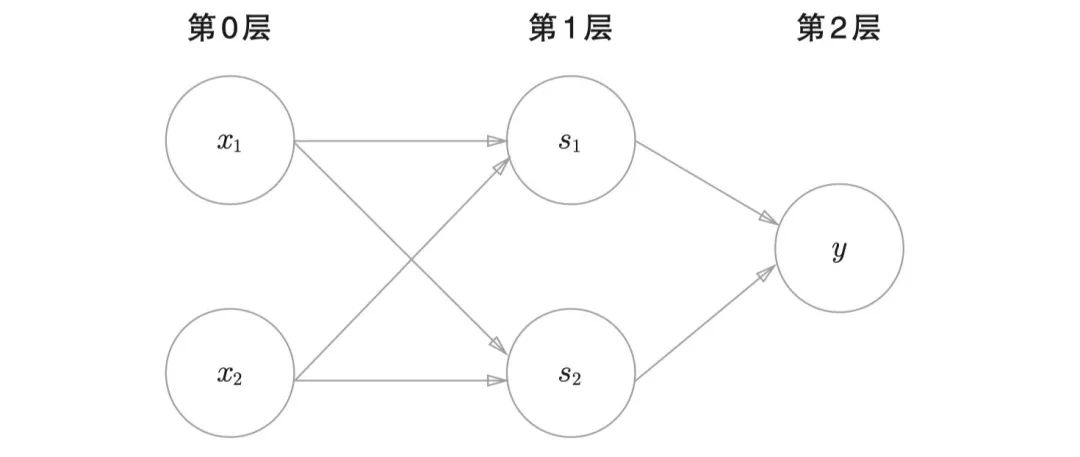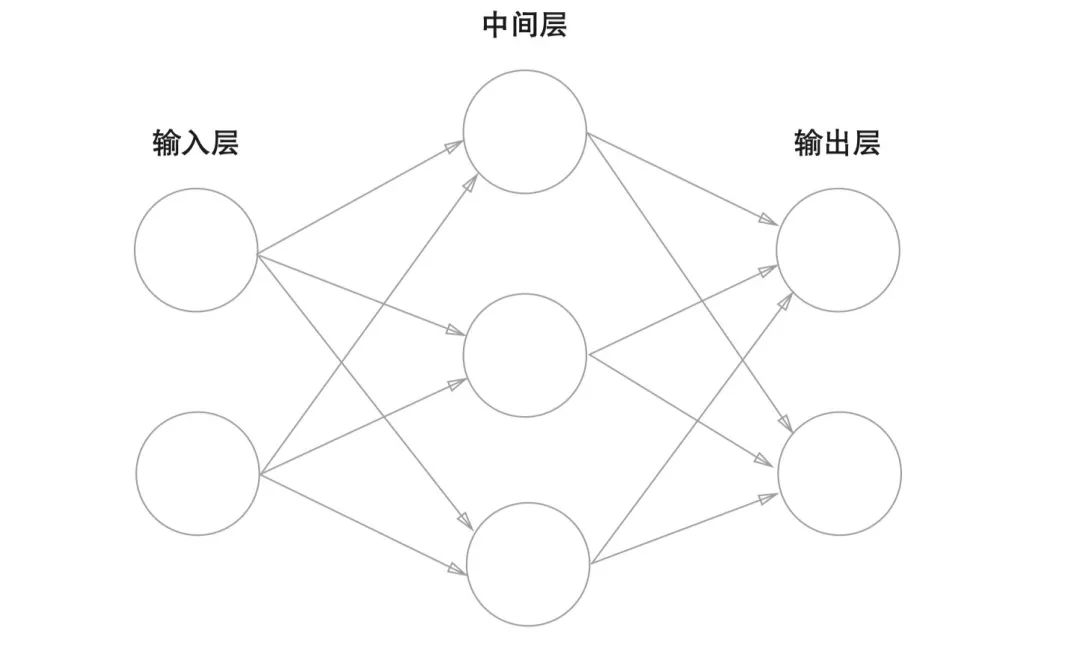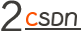### 信号传递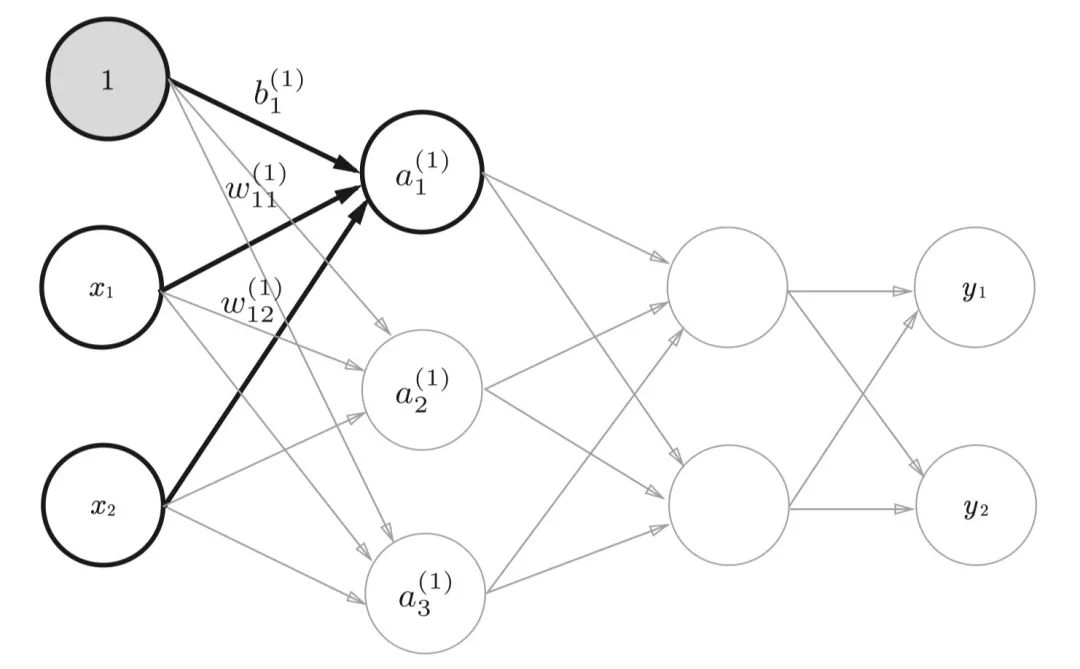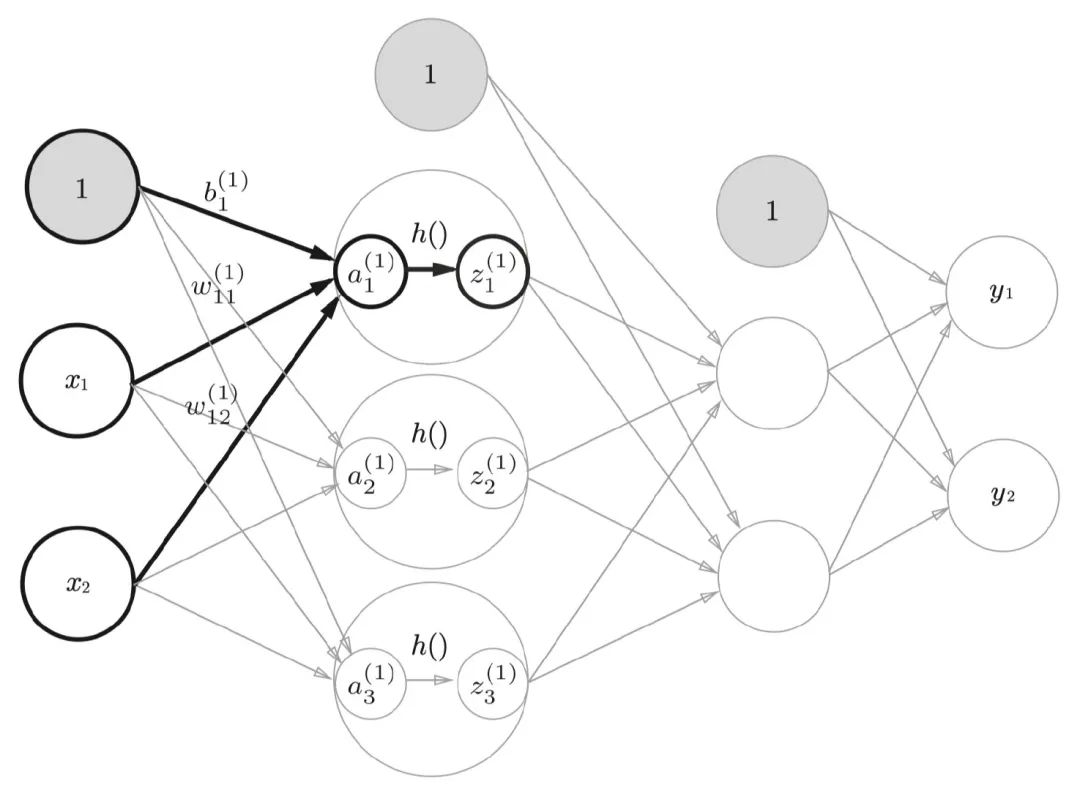Sigmoid我们已经很熟悉了，如果我们把LR模型也看成是一个单层的神经网络的话，那么Sigmoid就是它的激活函数。Sigmoid应用在二分类场景当中单个的输出节点上，输出的值 如果大于0.5表示为真，否则为假。在一些概率预估场景当中，也可以认为输出值就代表了事件发生的概率。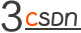### 代码实现

``````import numpy as np

def relu(x):
return np.where(x > 0, x, 0)

def sigmoid(x):
return 1 / (1 + np.exp(-x))

class NeuralNetwork():
def __init__(self):
self.params = {}
self.params['W1'] = np.random.rand(2, 3)
self.params['b1'] = np.random.rand(1, 3)
self.params['W2'] = np.random.rand(3, 2)
self.params['b2'] = np.random.rand(1, 2)
self.params['W3'] = np.random.rand(2, 1)
self.params['b3'] = np.random.rand(1, 1)

def forward(self, x):
a1 = np.dot(x, self.params['W1']) + self.params['b1']
z1 = relu(a1)

a2 = np.dot(z1, self.params['W2']) + self.params['b2']
z2 = relu(a2)

a3 = np.dot(z2, self.params['W3']) + self.params['b3']
return np.where(sigmoid(a3) > 0.5, 1, 0)

if __name__ == "__main__":
nn = NeuralNetwork()
print(nn.forward(np.array([3, 2])))
``````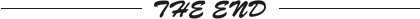``````更多精彩推荐
☞常年“盘踞”数据库前五的 MongoDB，在中国有哪些新动向？
☞开发者实测 M1 芯片报告：除了大型应用程序启动慢点，整体性能优秀

☞她们，在字节跳动写代码
☞“跟风离职后，找不到工作了！”：好多同事离职，这家公司还值不值得待？
☞中招！330 万台老年机被植木马，背后黑幕细思极恐

☞Salesforce 为什么要收购 Slack？
☞中台“不火”了，企业数智转型如何破圈？

``````20年前，《新程序员》创刊时，我们的心愿是全面关注程序员成长，中国将拥有新一代世界级的程序员。20年后的今天，我们有了新的使命：助力中国IT技术人成长，成就一亿技术人！